Next: Pulse Propagation in Two Up: Dispersive Waves Previous: Electromagnetic Wave Propagation in

# Waveguides

As we saw in Section 7.5, transmission lines (e.g., ethernet cables) are used to carry high frequency electromagnetic signals over distances that are long compared to the signal wavelength,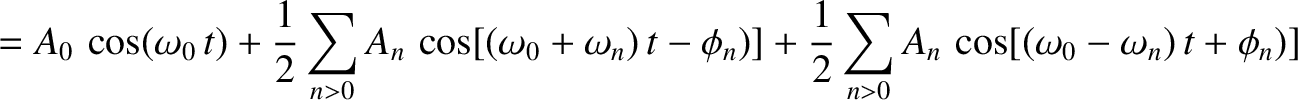, where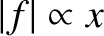is the velocity of light and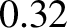the signal frequency (in hertz). Unfortunately, conventional transmission lines are subject to radiative losses (because the lines effectively act as antennas) which increase as the fourth power of the signal frequency (Fitzpatrick 2008). Above a certain critical frequency, which typically lies in the microwave band, the radiative losses become intolerably large. Under these circumstances, the transmission line must be replaced by a device known as a waveguide. A waveguide is basically a long hollow metal box within which electromagnetic signals propagate. Provided the walls of the box are much thicker than the skin-depth (see Section 10.4) in the wall material, the signal is essentially isolated from the outside world, and radiative losses are consequently negligible.

Consider an evacuated waveguide of rectangular cross-section that runs along the-direction, and is enclosed by perfectly conducting (i.e., infinite conductivity) metal walls located at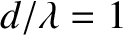,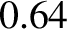,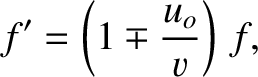, and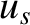. Suppose that an electromagnetic wave propagates along the waveguide in the-direction. For the sake of simplicity, let there be no-variation of the wave electric or magnetic fields. The wave propagation inside the waveguide is governed by the two-dimensional wave equation [cf., Equation (537)]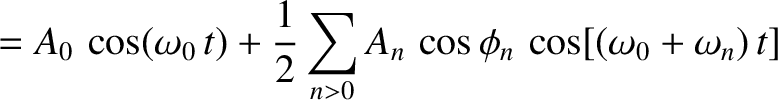(883)

where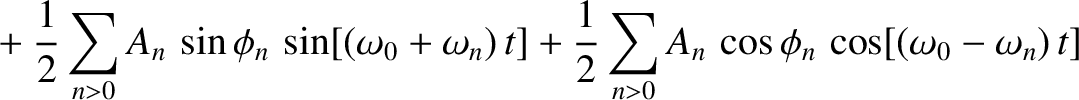represents the electric component of the wave, which is assumed to be everywhere parallel to the-axis, andis the velocity of light in vacuum. The appropriate boundary conditions are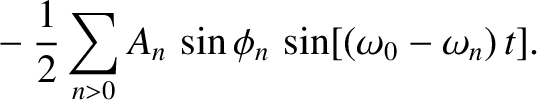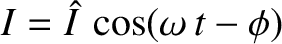(884)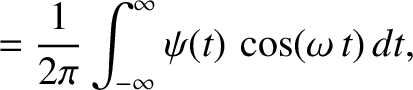(885)

because the electric field inside a perfect conductor is zero (otherwise, an infinite current would flow), and, according to standard electromagnetic theory (see Appendix C), there cannot be a tangential discontinuity in the electric field at a conductor/vacuum boundary. (There can, however, be a normal discontinuity. This permits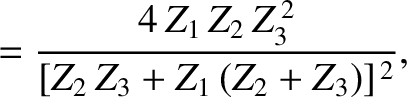to be non-zero atand.)

Let us search for a separable solution of Equation (883) of the form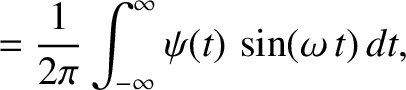(886)

whererepresents the-component of the wavevector (rather than its magnitude), and is the effective wavenumber for propagation along the waveguide. The previous solution automatically satisfies the boundary condition (884). The second boundary condition (885) is satisfied provided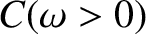(887)

where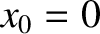is a positive integer. Suppose thattakes its smallest possible value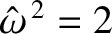. (cannot be zero, because, in this case,everywhere.) Substitution of expression (886) into the wave equation (883) yields the dispersion relation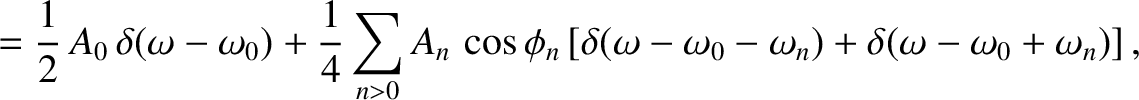(888)

where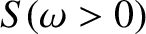(889)

This dispersion relation is analogous in form to the dispersion relation (797) for an electromagnetic wave propagating through a plasma, with the cut-off frequency,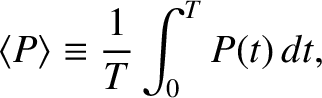, playing the role of the plasma frequency,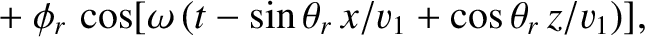. The cut-off frequency is so-called because for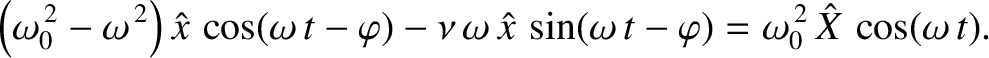the wavenumber is imaginary (i.e.,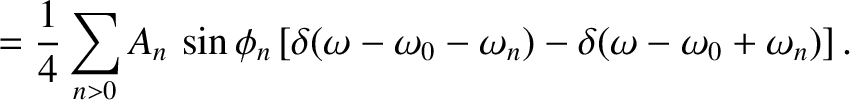), which implies that the wave does not propagate along the waveguide, but, instead, decays exponentially with increasing. On the other hand, for wave frequencies above the cut-off frequency the phase velocity,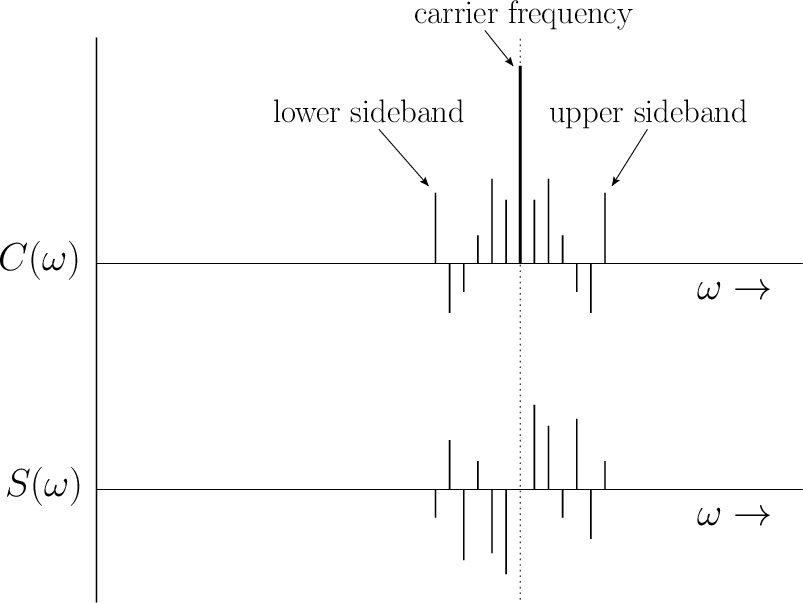(890)

is superluminal. This is not a problem, however, because the group velocity,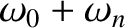(891)

which is the true signal velocity, remains sub-luminal. (Recall, from Section 10.2, that a high frequency electromagnetic wave propagating through a plasma exhibits similar behavior.) Not surprisingly, the signal velocity goes to zero as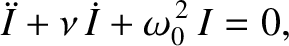, because the signal ceases to propagate at all when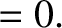.

It turns out that waveguides support many distinct modes of propagation. The type of mode discussed previously is termed a TE (for transverse electric-field) mode, because the electric field is transverse to the direction of propagation. (See Exercise 12.) There are many different sorts of TE mode, corresponding, for instance, to different choices of the mode number,(Fitzpatrick 2008). However, the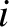mode has the lowest cut-off frequency. There are also TM (for transverse magnetic-field) modes (see Exercise 13), and TEM (for transverse electric- and magnetic-field) modes (ibid.). TM modes also only propagate when the wave frequency exceeds a cut-off frequency. On the other hand, TEM modes (which are the same type of mode as that supported by a conventional transmission line) propagate at all frequencies. However, TEM modes are only possible when the waveguide possesses an internal conductor running along its length (ibid.).Next: Pulse Propagation in Two Up: Dispersive Waves Previous: Electromagnetic Wave Propagation in
Richard Fitzpatrick 2013-04-08